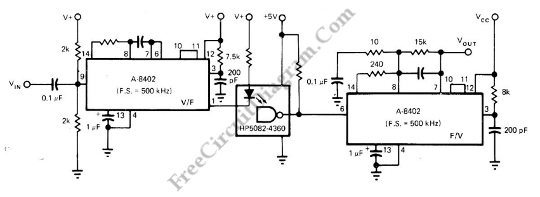# 30-Khz Bandwidth High Linearity Opto-Isolated AmplifierUsing optocoupler to directly transfer a linear (analog) voltage would produce  a large unpredictable linearity error, which is also sensitive to temperature changes. By using frequency modulation, the total system’s linearity will  depend only on the linearity of the V/F and F/V converter, which is fortunately cancel each other if we use similar devices with similar non-linearity error characteristic. A Intech/Function Modules A-8402 voltage-to-frequency converter is used to build isolation amplifier circuit. Here is the schematic diagram of the circuit:This  Intech/Function Modules A-8402 voltage-to-frequency converter has linearity of ±05%. It can convert input voltage to proportional frequency up to 500 kHz. Then this frequency will be used to drive the LED of opto-isolator.  The output of the optoisolator will be converted back proportional DC voltage by the similar IC. The converter need supply voltage of 12V, but it can be 5 to 18V.